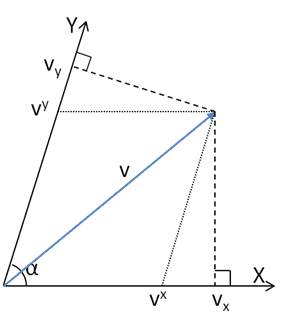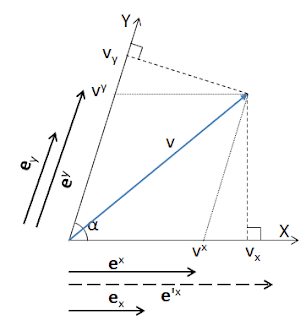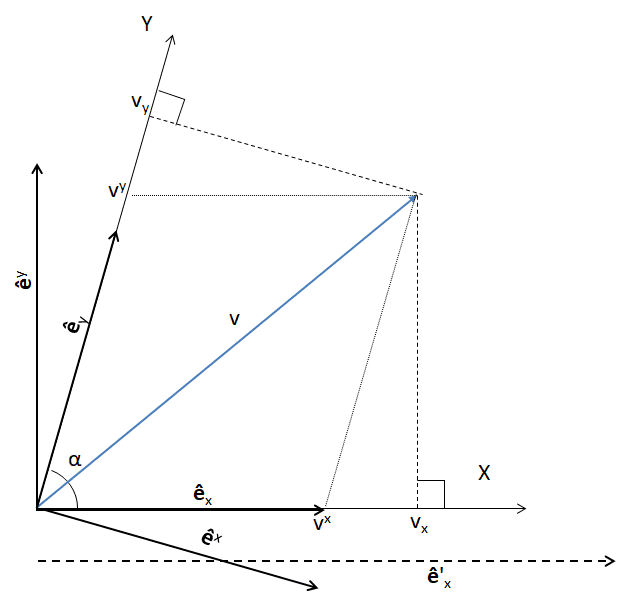# Covariant coordinates don't co-vary

Gold Member

## Homework StatementI am studying co- and contra- variant vectors and I found the video at youtube.com/watch?v=8vBfTyBPu-4 very useful. It discusses the slanted coordinate system above where the X, Y axes are at an angle of α. One can get the components of v either by dropping perpendiculars to the axes (vi) or by dropping a line parallel to the other axis (vi). These give correct results for the norm vivi and the dot product vi wi. (I have not shown w). So the vi are called contravariant and the vi are called covariant. According to the video Dirac thought this was a great example.

But both vi and vi contra-vary with a change of scale of the basis vectors. This contradicts some definitions of contravariant and covariant components, e.g. this one on Wikipedia. These definitions say that covariant components co-vary with a change of scale.

Is there a simple resolution to this apparent contradiction?

## Homework Equations

Norm and dot product were calculated by expressing vi and vi in Cartesian coordinates. Quite a lot of equations! Along the way we found the metric for the slanted coordinate system.

## The Attempt at a Solution

We can demonstrate the problem by drawing basis vectors on the diagram and then another set double their size.

#### Attachments

•JD_PM

Staff Emeritus
Homework Helper
Gold Member
The covariant and contravariant components belong to different basis vectors.

•JD_PM, George Keeling and Cryo
Gold Member
Why not think about vectors in terms of partial derivatives? You have space, which you can label with some coordinates ##\left\{x^{(1)}, x^{(2)}, \dots\right\}##. Imagine there is a differentiable, scalar function ##f=f\left(x^{(1)}, x^{(2)}, \dots\right)## defined everywhere in space. Consider the difference of the value of function between two closely-spaces points

##df=f\left(x^{(1)}+a^{1},\dots\right)-f\left(x^{(1)},\dots\right)##, where ##a^{\dots}\to 0##

Keeping only first order terms the result is:

##df=a^i \partial_i f + \mathcal{O}\left(a^2\right)##

Clearly the difference ##df## will not depend on the coorinate system. You can compute how the partial derivatives ##\partial_i f=\frac{\partial f}{\partial x^{(i)}}## change when you change the coordinates. It follows that ##a^i## must change in the 'opposite' way, to ensure invariance of ##df##: that's your contra-variant vector. The rules for changing the co-variant vectors, i.e. ##w_i##, follow from the fact that ##w_i a^i = scalar##, since ##w_i## is a linear functional over the vector space of contra-variant vectors, i.e. by definition ##w_i a^i = scalar##

•JD_PM and George Keeling
Gold Member
Thank you Orodruin. Cryo is a bit harder. Following Orodruin I immediately saw that I needed smaller covariant basis vectors which I call ex, ey. I realize that the up/down position of the indices is debatable. Now I have a new diagram showing a few of the basis vectors.To get v with the covariant coordinates we must have
v = vxex + vyey . . . . . . . (1)​
I have drawn them in on the diagram and ex is clearly smaller than ex, which I have conveniently drawn so that vx = 1. We must also have
vxex = vxex . . . . . . . (2)​
and similarly
vyey = vyey . . . . . . . (3)​
which give us
v = vxex + vyey . . . . . . . (4)​
which is as it should be.
(3) gives us
vy = ey(ey)-1vy . . . . . . . (5)​
where (ey)-1 is the inverse of ey: or ey(ey)-1 = 1.
(5) shows us that vy does indeed vary with ey with a strange constant of proportionality (ey)-1vy.

PS Is there a way to do hard spaces in BB Code? I couldn't see anything in https://www.physicsforums.com/help/bb-codes.

#### Attachments

Last edited:
Staff Emeritus
Homework Helper
Gold Member
Your diagram is not correct. Generally the dual basis is not parallel to the tangent basis (it is orthogonal to the coordinate surfaces, not tangent to the coordinate lines).

•George Keeling
Gold Member
Orodruin:
Is it true, like contravariants, that for covariant bases / coordinates

v = vxex + vyey

Staff Emeritus
Homework Helper
Gold Member
A vector can be written in terms of any basis with the corresponding components. When you have a coordinate system with coordinate functions ##x^a## you can introduce the tangent basis ##\vec e_a = \partial \vec x/\partial x^a## and the dual basis ##\vec e^a = \nabla x^a##, respectively. The contravariant components by definition are those components that express a vector in the tangent basis and the covariant components by definition are those that express a vector in the dual basis.

You can write ##\vec v = v^a \vec e_a## or ##\vec v = v_a \vec e^a##, but generally this is not the same as ##v_a\vec e_a##, which does not make sense because you are then creating a vector that has the same contravariant components as the covariant components of ##\vec v## in this particular coordinate system.

•JD_PM, vanhees71 and George Keeling
Gold Member
I did say
covariant basis vectors which I call ex, ey. I realize that the up/down position of the indices is debatable.
. In that context the answer to my question
v = vxex + vyey
is yes because (positioning indices less debatably)
##\vec v = v_a \vec e^a##
.
Now that I have learned that ##\vec e^a = \nabla x^a##, it should be easy to make progress. (Famous last words)

Gold Member
I think the other contributors already explained the significance of derivatives when talking about vectors on manifolds, which is what you are doing. I will just add one more thing about the dual space.

As I said in my earlier post one can introduce the space of contra-variant vectors ##v^i## through partial derivatives. What was confusing for me, when I was learning, is how to think about the dual space, i.e. ##v_i##. Sometimes people say that if ##v^i## is a vector then ##v_i## is like a surface through which this vector (field) ##v^i## flows. I do not quite like this (e.g. see Bachman "Geometric approach to differential forms" -> Sec. 5.7 "How not to visualize a differential 1-from"). What I have come to realize is that the best way to think about dual space is as functionals - which is the normal way do define it (e.g. Halmos "Finite-dimensional vector spaces").

I.e. you define your contra-variant vector space ##V## whichever way you like (as long as it makes sense). Then the dual space is a space ##V^*## of linear functions ##\mathbf{f}: V \to \mathbb{R}## (or ##\mathbb{C}##), i. e. if ##\mathbf{v}, \mathbf{w} \in V##, ##\alpha, \beta \in \mathbb{R}## (or ##\mathbb{C}##) and ##\mathbf{f} \in V^*## then:

##\mathbf{f}\left(\alpha\mathbf{v} + \beta\mathbf{w}\right) = \alpha \mathbf{f}\left(\mathbf{v} \right) + \beta \mathbf{f}\left(\mathbf{w} \right) = real\, number## (or ##complex\, number##)

This may be a weird way of looking at it, but it makes it plainly obvious that the dual space ##V^*## is different from ##V##. How do you square this with the conventional notions? Let's say your basis for (finite-dimensional) ##V## is ##\mathbf{e}_i##, i.e. ##\mathbf{v} \in V \implies \mathbf{v}=v^i\mathbf{e}_i##. Then you could introduce a basis-set of functionals ##\mathbf{q}^i: V\to \mathbb{R}## such that:

##\mathbf{q}^i \left(\mathbf{e}_j\right) = \delta^i_j =
\begin{cases}
1, & \text{if $i=j$}.\\
0, & \text{otherwise}.
\end{cases}
##

Then any member of dual space ##\mathbf{f} \in V^*## is given by ##\mathbf{f}=f_i \mathbf{q}^i##, and:

##f_i v^i = \mathbf{f}\left(\mathbf{v}\right) = f_i v^j \mathbf{q}^i \left(\mathbf{e}_j\right) = f_i v^j \delta^i_j##

Last edited:
•George Keeling
Gold Member
Thinking of dual space as of space of linear functions also explains why co-variant vectors must co-vary, since ##\mathbf{f}\left(\mathbf{v}\right)=scalar## is a statement that does not depend on coordinates, so that ##scalar## has to be the same irrespective of the coordinte system and basis sets used to span the vector and dual vector spaces.

Gold Member
Following Orodruin's great tip
##ê^j = \nabla x^j##
(I am using ^ instead of → over basis vectors, after Sean Carroll.) Since I already knew the inverse metric of the skewed system, it was easy to calculate that
$$ê^1=\hat x - \frac { \hat y } {\tan \alpha} \\ {\rm and} \phantom {10000000000000000000000000000000000000000000000000000000000}\\ ê^2=\frac { \hat y } {\sin \alpha}$$ where ##\hat x, \hat y## are the Cartesian basis vectors. In can now redraw my diagram (##x=1,y=2##)##ê^x## is ##\pi / 2 - \alpha## below the Cartesian X axis. Therefore the angle between ##ê_y## and ##ê^x## is a right angle, and the projected line from ##v_y## is parallel to ##ê^x## as I should have known and Orodruin intimated when he wrote in #5 "the dual basis is ... orthogonal to the coordinate surfaces, not tangent to the coordinate lines". Likewise ##ê^y## is parallel to the projected line from ##v_x## and always on the Cartesian Y axis. In addition ##|ê^i |>|ê_i |##.

All this did not answer my original question that "both ##v^i## and ##v_i## contra-vary with a change of scale of the basis vectors". I had to use the pullback operator to find the metric of the primed system and then showed that if ##ê'_x=aê_x## and ##ê'_y=bê_y## (##a=2## in the diagram above) then
$$v'_x=a^2v'^x+abv'^y \cos \alpha\\ {\rm and} \phantom {10000000000000000000000000000000000000000000000000000000000}\\ v'_y=b^2v'^y+abv'^x \cos \alpha$$ Which does answer the question - the transformed covariant coordinates do covary with the contravariant basis vectors.

I have obviously left out quite a bit of detail. It is elsewhere. Thanks Dirac and PF and please like this post if I am correct.

Last edited:

## Homework Statement

View attachment 233849
I am studying co- and contra- variant vectors and I found the video at youtube.com/watch?v=8vBfTyBPu-4 very useful. It discusses the slanted coordinate system above where the X, Y axes are at an angle of α. One can get the components of v either by dropping perpendiculars to the axes (vi) or by dropping a line parallel to the other axis (vi). These give correct results for the norm vivi and the dot product vi wi. (I have not shown w). So the vi are called contravariant and the vi are called covariant. According laser to the video Dirac thought this was a great example.

But both vi and vi contra-vary with a change of scale of the basis vectors. This contradicts some definitions of contravariant and covariant components, e.g. this one on Wikipedia. These definitions say that covariant components co-vary with a change of scale.

Is there a simple resolution to this apparent contradiction?

## Homework Equations

Norm and dot product were calculated by expressing vi and vi in Cartesian coordinates. Quite a lot of equations! Along the way we found the metric for the slanted coordinate system.

## The Attempt at a Solution

We can demonstrate the problem by drawing basis vectors on the diagram and then another set double their size.
About the video you posted i have a question guys wouldn't the metric tensor need to be multiplied by 3 differentials since we're in 3-space? It seems like it would need dx dy dz. Can the metric tensor manipulate only dx and dy to move through 3-space? Not sure I understand.

Gold Member
I re-watched that video but only part 1. The first five minutes are about any number of dimensions and we saw things like the metric equation ##ds^2=g_{ij}dx^idy^j## which is using the Einstein summation convention and ##i,j## run from ##0...(n-1)## or ##1...n## where ##n## is the number of dimensions. In the second half it is only talking about two dimensions, (Euclidean or oblique). In that case in Euclidean ##dx^1=dx , dx^2=dy## and there is no ##dx^3## which would have been ##dz##. The metric equation in full is$$ds^2=g_{11}dxdx+g_{12}dxdy +g_{21}dydx +g_{22}dydy$$Also the metric is always symmetric so ##g_{12}=g_{21}##
Hope that helps!

••